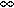# Statistical Theory of Fission Chains and Generalized Poisson Neutron Counting Distributions

Nuclear Science and Engineering / Volume 172 / Number 3 / November 2012 / Pages 300-326

Technical Paper / dx.doi.org/10.13182/NSE11-86

The neutron counting probability distribution for a multiplying medium was shown by Hage and Cifarelli to be a generalized Poisson distribution that depends on the fission chain number distribution. An analytic formula is obtained for this number distribution, the probability to produce a number of neutrons in a fission chain. The formula depends on the probability that a fission spectrum neutron induces a subsequent fission and depends on the probability distribution for a specific number of neutrons to be produced in an individual induced fission. The formula is an exact solution to a functional equation due to Böhnel for the probability generating function. The Böhnel equation is derived as the t [right arrow]limit of a rate equation for a neutron population generating function, related to a rate equation studied by Feynman. The Böhnel equation is also shown to be a fixed point of an iteration problem, related to one studied by Hawkins and Ulam, where the iteration generates the chain a generation at a time. The discrete iteration problem is shown to be connected to the continuous time evolution of the chain. An explicit solution for the time evolution of the chain is given in the simplified approximation where at most two neutrons are created by an induced fission. The t [right arrow]limit of this equation gives a simple analytic expression for the solution to the Böhnel equation in this approximation. A generalized Poisson counting distribution constructed from the theoretical fission chain probability number distribution is compared to experimental data for a multiplying Pu sample.